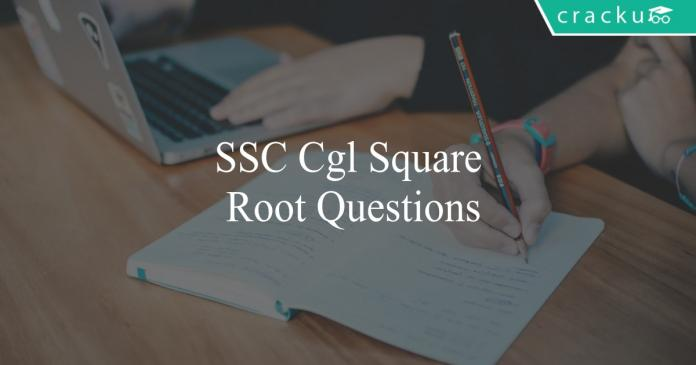# SSC CGL Square Root Questions PDF

0
2650ssc cgl square root questions

# SSC CGL Square Root Questions:

Download SSC CGL Trignometry questions with answers PDF based on previous papers very useful for SSC CGL exams. 20 Very important Trignometry objective questions for SSC exams.

Question 1: What is the positive square root of $[19+4\sqrt21]?$

a) $\sqrt{7}+2\sqrt{3}$

b) $\sqrt{3}+2\sqrt{7}$

c) $\sqrt{2}+3\sqrt{7}$

d) $\sqrt{7}+3\sqrt{3}$

Question 2: What is the square root of $\frac{(3-2\sqrt2)}{(3+2\sqrt2)}$ ?

a) $3-2\sqrt2$

b) $3+2\sqrt2$

c) $1$

d) $17$

Question 3: What is the square root of $\frac{(3-2\sqrt2)}{(3+2\sqrt2)}?$

a) $3-2\sqrt2$

b) $3+2\sqrt2$

c) $1$

d) 17$Question 4: Determine the value of m for which$4x+\frac{\sqrt{x}}{6}+\frac{m^2}{4}$is a perfect square. a)$\frac{1}{24}$b)$\frac{1}{12}$c)$12$d)$24$Question 5: What is the positive square root of$[25+4\sqrt{39}]$? a)$\sqrt{13}+2\sqrt3$b)$\sqrt{13}+3\sqrt2$c)$\sqrt{11}+2\sqrt3$d)$11+3\sqrt2$Question 6: Divide 20 into two parts such that the sum of the square of the parts is 232. What is the value of the two parts? a) 6, 14 b) 8, 12 c) 4, 16 d) 10, 10 Question 7: A triangle is inscribed inside a square such that the base of the triangle coincides with the side of the square. The square is inscribed inside a circle of diameter$24\sqrt{2}$cm. Then find the area of the triangle. a) 196 sq.cm b) 169 sq.cm c) 225 sq.cm d) 288 sq.cm Question 8: Find the area of a square which is inscribed in a circle of radius$7\sqrt{2}$cm. a) 144 sq.cm b) 196 sq.cm c) 289 sq.cm d) 49 sq.cm Question 9: The sum of two positive numbers is 20% of the sum of their squares and 25% of the difference of their squares. If the numbers are x and y the,$\ \frac{x+y}{x^{2}}\ $is equal to a)$\frac{1}{4}$b)$\frac{3}{8}$c)$\frac{1}{3}$d)$\frac{2}{9}$Question 10: What is the volume (in cm$^{3}$) of a right pyramid of height$12$cm and having a square base whose diagonal is$6\sqrt{2}$cm? a) 864 b) 432 c) 144 d) 288 Answers & Solutions: 1) Answer (A) Expression :$[19+4\sqrt21]?$=$[19+2\sqrt{4\times21}]=[19+2\sqrt{84}]$=$7+12+2\sqrt{7\times12}$=$(7)^2+(12)^2+2\sqrt{7\times12}$Now, we know that$a^2+b^2+2ab=(a+b)^2$=$(\sqrt{7}+\sqrt{12})^2$Thus, square root is =$\sqrt{7}+\sqrt{12}$=$\sqrt7+2\sqrt3$=> Ans – (A) 2) Answer (A) Given :$x=\frac{(3-2\sqrt2)}{(3+2\sqrt2)}$To find :$\sqrt{x}$Solution : rationalizing the denominator, we get =>$\frac{(3-2\sqrt2)}{(3+2\sqrt2)}\times\frac{(3-2\sqrt2)}{(3-2\sqrt2)}$=$\frac{(3-2\sqrt2)^2}{(3)^2-(2\sqrt2)^2}$=$\frac{(3-2\sqrt2)^2}{9-8}$=>$x=(3-2\sqrt2)^2$Taking square root on both sides, =>$\sqrt{x}=3-2\sqrt2$=> Ans – (A) 3) Answer (A) Expression :$\frac{(3-2\sqrt2)}{(3+2\sqrt2)}$Rationalizing the denominator, =$\frac{(3-2\sqrt2)}{(3+2\sqrt2)}\times\frac{(3-2\sqrt2)}{(3-2\sqrt2)}$=$\frac{(3-2\sqrt2)^2}{(3+2\sqrt2)(3-2\sqrt2)}$=$\frac{(3-2\sqrt2)^2}{9-8}=(3-2\sqrt2)^2$Thus, square root is =$3-2\sqrt2$=> Ans – (A) 4) Answer (B) Expression :$4x+\frac{\sqrt{x}}{6}+\frac{m^2}{4}$Let$\sqrt{x}=y$=$4y^2+\frac{y}{6}+\frac{m^2}{4}$=$(2y)^2+2(2y)(\frac{1}{24})+(\frac{m}{2})^2$Using,$a^2+2ab+b^2=(a+b)^2$=>$\frac{m}{2}=\frac{1}{24}$=>$m=\frac{2}{24}=\frac{1}{12}$=> Ans – (B) 5) Answer (A) Expression :$[25+4\sqrt{39}]$=$[25+2\sqrt{4\times39}]=[25+2\sqrt{156}]$=$13+12+2\sqrt{13\times12}$=$(13)^2+(12)^2+2\sqrt{13\times12}$Now, we know that$a^2+b^2+2ab=(a+b)^2$=$(\sqrt{13}+\sqrt{12})^2$Thus, square root is =$\sqrt{13}+\sqrt{12}$=$\sqrt13+2\sqrt3$=> Ans – (A) 6) Answer (A) Let the first part =$x$and second part =$(20 – x)$According to ques, =>$(x)^2 + (20 – x)^2 = 232$=>$x^2 + (x^2 + 400 – 40x) = 232$=>$2x^2 – 40x + 400 – 232 = 0$=>$x^2 – 20x + 84 = 0$=>$x^2 – 6x – 14x + 84 = 0$=>$x(x-6) – 14(x-6) = 0$=>$(x-6)(x-14) = 0$=>$x = 6,14$=> Ans – (A) 7) Answer (D) Diameter of the circle =$24\sqrt{2}$cm = Diagonal of the square. We know that, Diagonal of the square =$\sqrt{2}a$cm where a is side of the square.$\sqrt{2}a$=$24\sqrt{2}$⇒ a = 24 cm Here, Side of the square = Base of the triangle = Height of the triangle = 24 cm Therefore, Area of the triangle$= \dfrac{1}{2} \times \text{Base} \times \text{Height} = \dfrac{1}{2} \times 24 \times 24 = 288$sq.cm 8) Answer (B) Given that the radius of the circle =$7\sqrt{2}$cm. Then, the diameter of the circle =$14\sqrt{2}$cm. Here, Diameter of the circle = Diagonal of the square =$14\sqrt{2}$cm. We know that, Diagonal of the square =$\sqrt{2}a$cm where a is side of the square.$\sqrt{2}a$=$14\sqrt{2}$⇒ a = 14 cm Hence, Area of the square = 14*14 = 196 sq.cm 9) Answer (D) Given x+y$= \frac{20}{100}\times(x^{2}+y^{2})\Rightarrow$x+y$= \frac{1}{5}\times(x^{2}+y^{2})\Rightarrowx^{2}+y^{2} =$5(x+y)$\rightarrow$(1) Also Given x+y$= \frac{25}{100}\times(x^{2}-y^{2})\Rightarrow$x+y$= \frac{1}{4}\times(x^{2}-y^{2})\Rightarrowx^{2}-y^{2} =$4(x+y)$\rightarrow$(2) Adding equation(1) and equation(2)$\Rightarrow$2x$^{2} =$9(x+y)$\therefore\frac{x+y}{x^{2}} = \frac{2}{9}$10) Answer (C) Height of pyramid =$h=12$cm and diagonal of base =$d=6\sqrt2$cm Let side of square base =$s$cm =>$s^2+s^2=d^2$=>$2s^2=(6\sqrt2)^2=72$=>$s^2=\frac{72}{2}=36\therefore$Volume of pyramid =$\frac{1}{3}\times$Area of base$\times$Height =$\frac{1}{3}\times36\times12=144cm^3\$

=> Ans – (C)